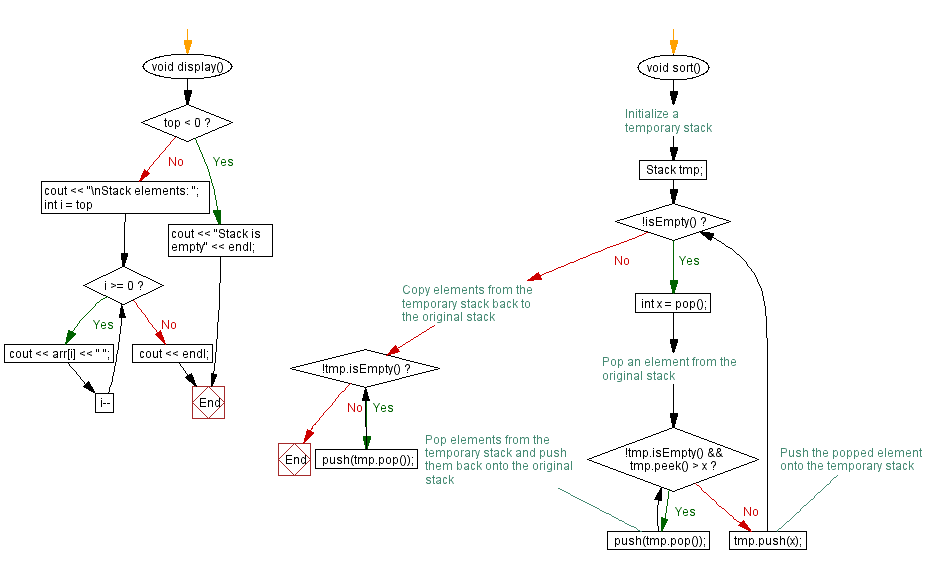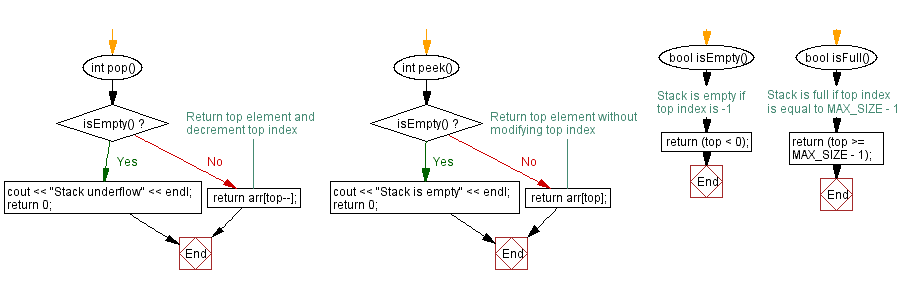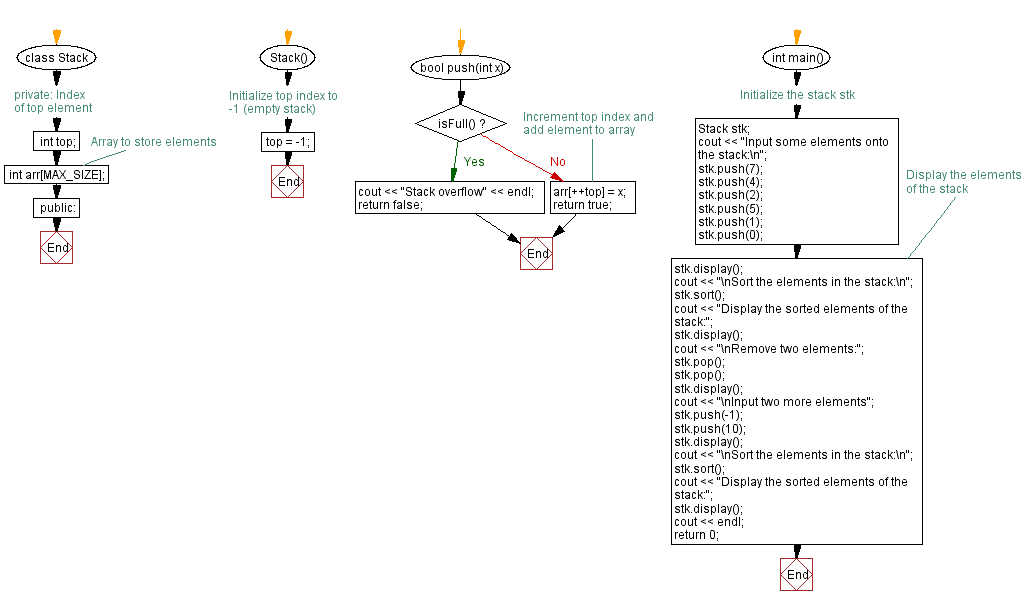﻿ C++ Sort a stack using another stack

# C++ Stack Exercises: Sort a stack using another stack

## C++ Stack: Exercise-3 with Solution

Write a C++ program to sort a given stack (using an array) using another stack.

Test Data:
Input some elements onto the stack:
Stack elements: 0 1 5 2 4 7
Sort the elements in the stack:
Display the sorted elements of the stack:
Stack elements: 0 1 2 4 5 7

Sample Solution:

C++ Code:

``````#include <iostream>

using namespace std;
#define MAX_SIZE 15 // Maximum size of stack

class Stack {
private:
int top; // Index of top element
int arr[MAX_SIZE]; // Array to store elements
public:
Stack() {
top = -1; // Initialize top index to -1 (empty stack)
}

bool push(int x) {
if (isFull()) {
cout << "Stack overflow" << endl;
return false;
}
// Increment top index and add element to array
arr[++top] = x;
return true;
}

int pop() {
if (isEmpty()) {
cout << "Stack underflow" << endl;
return 0;
}
return arr[top--];
}

int peek() {
if (isEmpty()) {
cout << "Stack is empty" << endl;
return 0;
}
return arr[top];
}

bool isEmpty() {
// Stack is empty if top index is -1
return (top < 0);
}

bool isFull() {
// Stack is full if top index is equal to MAX_SIZE - 1
return (top >= MAX_SIZE - 1);
}

void display() {
if (top < 0) {
cout << "Stack is empty" << endl;
return;
}
cout << "\nStack elements: ";
for (int i = top; i >= 0; i--)
cout << arr[i] << " ";
cout << endl;
}

void sort() {
// Initialize a temporary stack
Stack tmp;
while (!isEmpty()) {
int x = pop();
// Pop an element from the original stack
while (!tmp.isEmpty() && tmp.peek() > x) {
// Pop elements from the temporary stack and push them back onto the original stack
push(tmp.pop());
}
// Push the popped element onto the temporary stack
tmp.push(x);
}
// Copy elements from the temporary stack back to the original stack
while (!tmp.isEmpty()) {
push(tmp.pop());
}
}

};

int main() {
//Initialize the stack stk
Stack stk;
cout << "Input some elements onto the stack:\n";
stk.push(7);
stk.push(4);
stk.push(2);
stk.push(5);
stk.push(1);
stk.push(0);
// Display the elements of the stack
stk.display();
cout << "\nSort the elements in the stack:\n";
stk.sort();
cout << "Display the sorted elements of the stack:";
stk.display();
cout << "\nRemove two elements:";
stk.pop();
stk.pop();
stk.display();
cout << "\nInput two more elements";
stk.push(-1);
stk.push(10);
stk.display();
cout << "\nSort the elements in the stack:\n";
stk.sort();
cout << "Display the sorted elements of the stack:";
stk.display();
cout << endl;
return 0;
}
``````

Sample Output:

```Input some elements onto the stack:

Stack elements: 0 1 5 2 4 7

Sort the elements in the stack:
Display the sorted elements of the stack:
Stack elements: 0 1 2 4 5 7

Remove two elements:
Stack elements: 2 4 5 7

Input two more elements
Stack elements: 10 -1 2 4 5 7

Sort the elements in the stack:
Display the sorted elements of the stack:
Stack elements: -1 2 4 5 7 10
```

Flowchart:CPP Code Editor: